A recent Numberphile video inspired this post. To summarize the question I was interested in, can you find a set of three numbers that, when any pair of the three numbers are summed, they equal a power of two?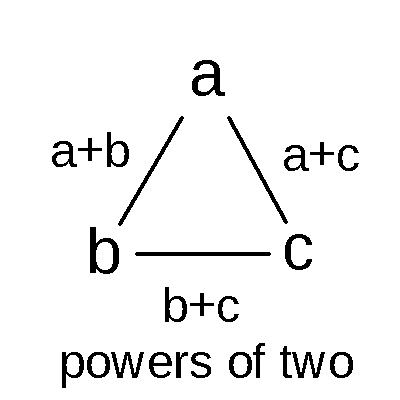It's a little intimidating to solve with three numbers, so let's start with two instead. Since the sum of the two numbers must be a power of 2, the average value of each number must be half of that power of 2. This lets us write the potential solutions in terms of the average.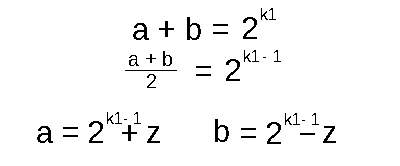Any value can be put into "z" leading to infinitely many solutions for a two number setup. Let's try with three now.

With just two numbers, there's only one way to sum them. With three numbers, there's three ways to sum them in pairs. So, let's define a k2 and k3 variable.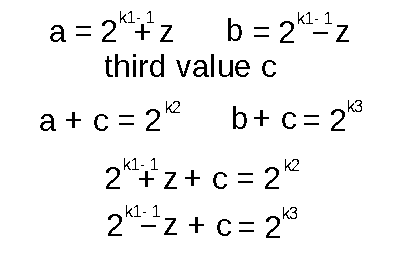After doing some substitution, we have a system of two equations to work with. With these, we are able to cancel out some terms and find out what z equals!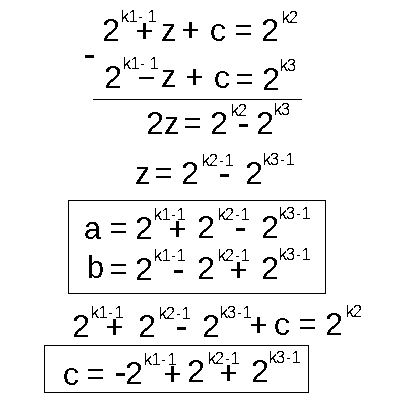Now the k variables are completely arbitrary, and can be redefined for the sake of making the equation shorter. We can shift each k variable by 1 to remove the "-1" next to each of them. This leaves up with the following set of solutions: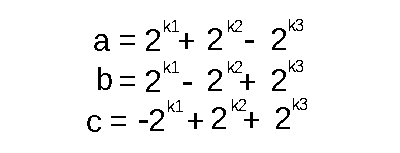There are a few interesting points to get out of this solution set. The first is that there MUST be exactly one negative number and two positive numbers. The second is that each number can be composed from three powers of two, with three free k variables where any positive integer can be used. This means there are infinitely many sets of three numbers with the properties we are looking for.

Here is an example solution that works: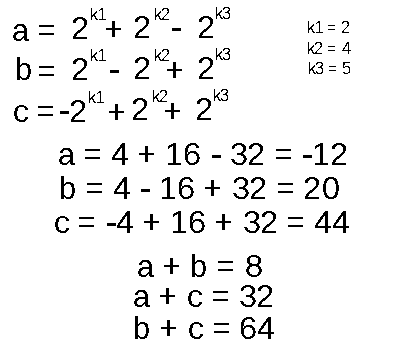Excellent. What about four variables? Can we come up with a solution set of 4 numbers? We will need 6 total pairs summing to a square number. Seems like a tough problem, but we do have three free variables to restrict. Additionally, we already have three out of four numbers that have all their pair sums to what we want. Just like removing any of the three variables gives us a valid two-number solution, removing one of four numbers of our hypothetical solution should get us something like what we already have here. So, let's introduce one more variable.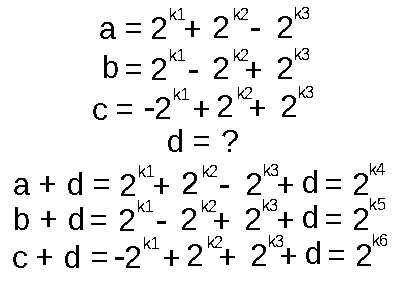We can again, subtract two equations to try and get some new info: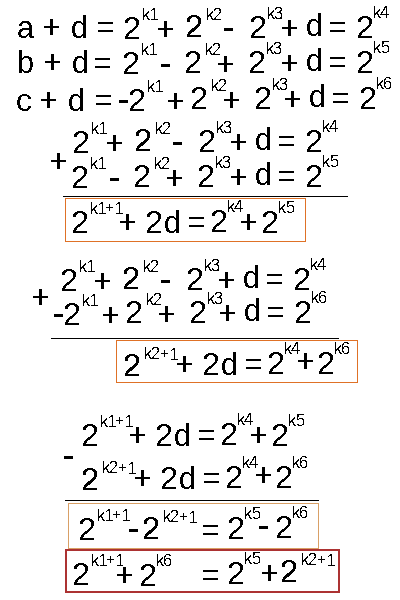This equation highlighted in red concerns me. Given any positive integer, there is only one way to write it in terms of unique powers of 2. This is the same principle as to why each positive integer has one representation in binary, or base 2. You can read each side of the equation as some number where in binary there are only two "1"s somewhere in the number, as each power of 2 sets a binary digit to 1 (there is a case where the two powers of two are the same value, so there'd effectively be just one "1" in the binary). Since each side of the equation must be the same, there are only a couple possibilities what the k variables must be: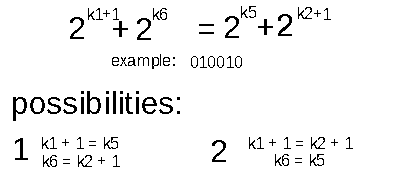I can tell you definitely that k1 + 1 = k2 + 1 is not going to give us any good solutions. Why is that? Well, the sums of pairs should all be unique. I didn't mention that, but it was implied.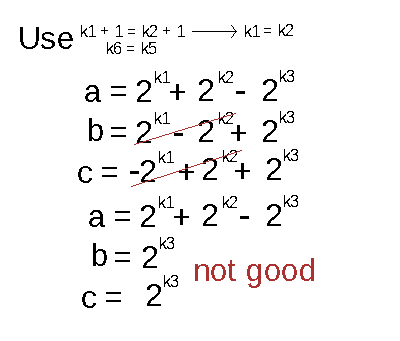As you can see, the sum of a + b will be the same as a + c, and we want unique sums of powers of two. So, this fails. But what about the other possibility?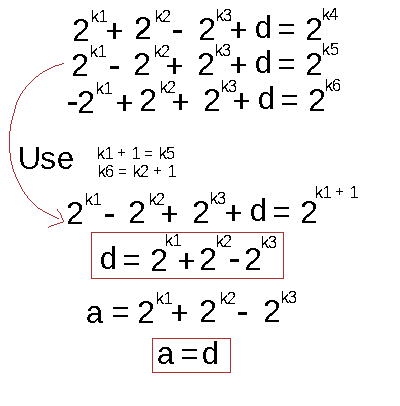d will be the same value as a! But that means we will get duplicate powers of two sums! (ex. a + b = d + b). Therefore, no such set of 4 numbers can give us all unique pair sums of powers of two. The best we can get is 4 out of 6 unique powers of two (a + b, a + c, b + c, a + d)